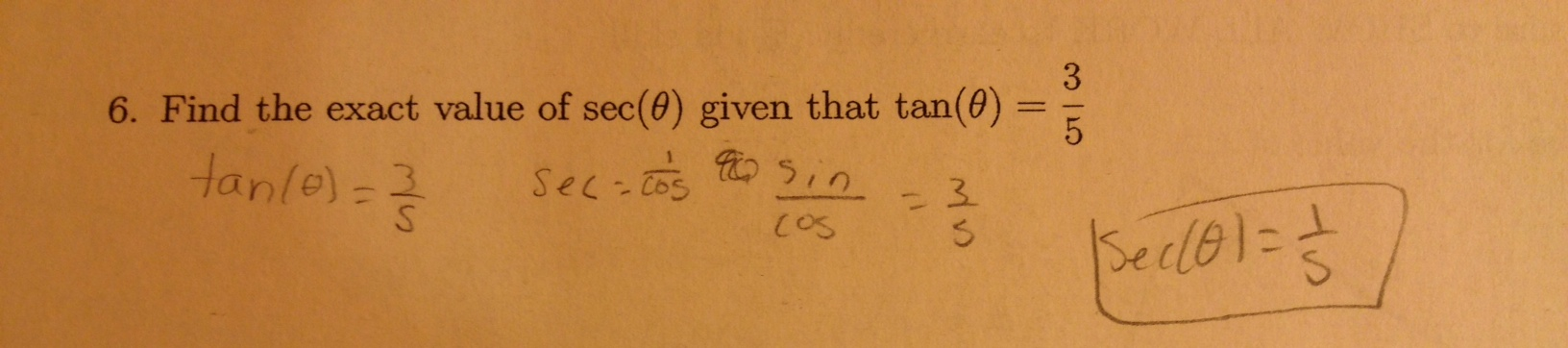Categories

# If sin(x)/cos(x) = 3/5, then 1/cos(x) = 1/5.The submitter asks: What would you write as feedback here? What would actually help this kid?

I ask: What’s the role of written feedback, more generally?

(Thanks again, Tina!)

## 9 replies on “If sin(x)/cos(x) = 3/5, then 1/cos(x) = 1/5.”

So many issues here: the idea that if x = a/b, and x = c/d, then c = a and b = d; that cos (theta) could ever be > 1; others I’m not smart enough to figure out.

But can I point out that that’s not a great question, because I’ve no idea what quadrant theta is in? Or is that being overly pedantic?

Good point about the quadrant. I will edit the question in case we use it again next year!

The quadrant is surely relevant, so it isn’t being pedantic to bring it up.

But if we knew we were operating in Q1, I would ask, “Is there a triangle here? If so, what do you know about it?” I think it’s easy to forget the context and get lost in algebraic manipulation mindlessly. And yes, asking how cosine (theta) could be > 1 is another good query.Bobsays:

The student seems to remember how the trig functions relate to one another but lacks conceptual understanding of what tan @ = 3/5 actual means with respect to right triangles. I would give the student a picture of the right triangle quadrant 1 with angle @ and then ask to find all 3 sides(and explain how they know) given that the tan @ = 3/5. How could you use this information to find sec @?sean mcbethsays:

seems likely to be a lapse in understanding of ratios. I would be curious to see algebraic ratios of n * y versus m * x from this student, for enumerated constant values of n and m and values of y and x that would violate the apparent ratio between n and m.sean mcbethsays:

and I mean the nature of ratios, that they are relationships between values, not values unto themselves.

As for actual written feedback to this kid, I’d write, “great hypothesis — I can follow your reasoning about how different trig functions are related” (because it is a great hypothesis). And then follow up with, “Can you draw and label the sides of a real triangle to show that your answer works i.e. where tan @ = 3/5 and sec @ = 1/5?”

That task acknowledges the kid’s thinking so far and teaches a general strategy to recover from this kind of tantalizing algebraic manipulation.

In the process of building that triangle, the kid is likely to encounter both where their thinking about proportions went wrong, and why the cosine is actually going to be less than one, and have the experience Bob outlines (but coming from the perspective of continuing their thinking by checking it vs. starting over with a new strategy).

If this were non-written feedback, I’d tend to ask, “great, how can we check that?” and be prepared to offer a strategy, but since checking is not most students’ strong suit, in written feedback I present the challenge right out, as a follow-up challenge. If the student is successful the next written prompt would be to reflect on why I asked them to draw and label a real triangle.

Good thoughts to chew on here. I wrote 3/5=6/10 on the paper of any kid who did this. I may have written 4=2+2 though. Kids all called me over and said “yeah, so?” Though it did get them to call me over so then I could have the conversation of fractions being about relationships, not values. I didn’t think to mention some of the great suggestions here, but asked students for a way to relate tangent to secant, they looked to their list of identities and found the relevant pythagorean one. Same end result as drawing the triangle but less conceptual understanding perhaps…

I call these “xyr” problems. If you know tan(theta) = y/x then you need r since sec(theta) = r/x. Then use x^2+y^2=r^2. This is simpler than the identities here,